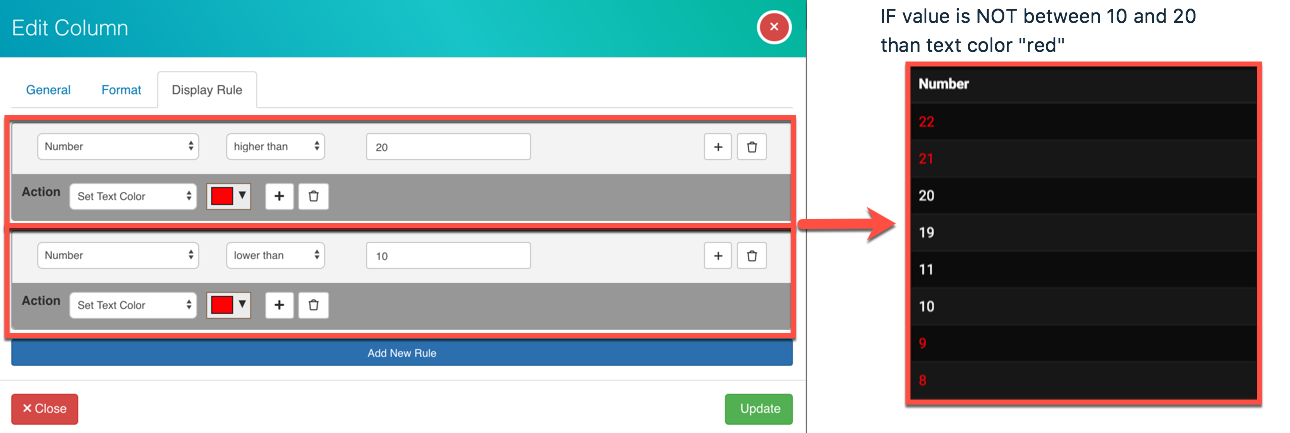Start a new topic
Implemented

## Having Rule : "Is NOT between X and Z Value X<>Z - Is Lower than Field A

Having new operator comparisons such as :

- IF value is  NOT between X and Z Values...

than text color "red"

- If Value of Field B is Higher than Field A...

Than text color "red"

For your first request you can do that by setting the rules displayed below.For your second request the only way I can think of accomplishing this as of now is by adding a  Basic Formula that subtracts Field A - Field B and then adding a display rule that checks to see if the formula is less than 0.

Field A = 20

Field B = 30

Basic Formula (A-B) = -10

IF Basic Formula is less than 0 then B is greater than A

Yes, thats exactly what I ve used for both case.

1) I though that case 1 could be joined in just one single line...whenever the operators <> would/could be agregated.

2) as for the 2nd case, I think that it implies an extra formula field appearing (also) on the form which is not always practical.
That is also the Matter of another Topic I wrotte about how to possibly start to benefit/use hidden (existing) fields in the form formulas ...without having to display some "live".... :)

:)